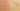ArithmeticPlusPrecedes

Misconception:

In a case like 1 + 2 + "AAA" + 3 + 4, first the numbers are added so we get 3 + "AAA" + 7, and then they are concatenated with the strings so we get "3AAA7".Incorrect

Addition has higher precedence than string concatenation

Correct

Addition and string concatenation have same precedence

CorrectionHere is what's right.

The arithmetic + operator has the same precedence as the string concatenation + operator. Syntactically, they really are the same operator. Moreover, their associativity is left to right.

So 1 + 2 + "AAA" + 3 + 4 evaluates to "3AAA34" as follows:

1 + 2 + "AAA" + 3 + 4
3 + "AAA" + 3 + 4
"3AAA" + 3 + 4
"3AAA3" + 4
"3AAA34"

The corresponding expression tree looks as follows. Note how, when proceeding from bottom up, the numbers are only added until the first + involving a string. From then on, there is only string-concatenation, because one of the operands always is a string.

OriginWhere could this misconception come from?

Students are familiar with arithmetics. The idea of operating on something different than numbers is less familiar to them. Thus they may go and evaluate those subexpressions they are familiar with, and postpone those (involving values of type String) until after that.

ValueHow can you build on this misconception?

We are not aware of any language that overloads the same operator (e.g., +) for arithmetic addition and string concatenation and uses different precedences for these two different interpretations of the operator. (Note that doing so would be complicated, because the grammar couldn’t easily distinguish between the two different uses of the operator given that they are represented by the exact same token.)

However, there are languages where string concatenation uses a different operator than arithmetic addition. E.g., PHP uses ., Lua uses .., and Ada uses & to concatenate strings.

When different operators are used, then they probably also have different precedences, and thus the arithmetic subexpressions may indeed be evaluated before their result is concatenated with the strings. Thus, this misconception actually is pretty valuable, because it represents the thinking in a “cleaner” world without operator overloading.

Java